# How changes to the numerator and the denominator impacts on the ratio

• Study Reminders

We'll email you at these times to remind you to study

You can set up to 7 reminders per week

#### You're all set

We'll email you at these times to remind you to study

Monday

Tuesday

Wednesday

Thursday

Friday

Saturday

Sunday

• Study Notes
• Discuss This Topic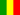Gabriel O. 1 0 In the calculation of a turnover ratio,the numeratoris an amount from an annual income statement,while the denominator is a balance sheet amount .Since a balance sheet amount is a snapshot and reflects only an instant or moment ,there is an inconsistency between the numerator and the denominator . However,the numerator in the inventory turnover ratio is the cost of goods sold for the 365 days in year, while the denominator reflects the cost of inventory for a just one moment at the end of the last day of the accounting year . To overcome this shortcomings,the denominator needs to be representative of all the moments during the year .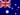Wendy C. 1 0 The net profit is the numerator and the total assets is the denominator.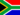Samuel F. 0 0 How do numerators and denominators effect the value of ratios?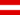Harrison A. 1 0 In the calculation of a turnover ratio,the numeratoris an amount from an annual income statement,while the denominator is a balance sheet amount .Since a balance sheet amount is a snapshot and reflects only an instant or moment ,there is an inconsistency between the numerator and the denominator . However,the numerator in the inventory turnover ratio is the cost of goods sold for the 365 days in year, while the denominator reflects the cost of inventory for a just one moment at the end of the last day of the accounting year . To overcome this shortcomings,the denominator needs to be representative of all the moments during the year .Harrison A. 0 0 what are the benefits of these calculation ?Zachary B. 0 0 What is accounting information?Diamond T. 0 0 Higher numerator => larger value of fraction (and vice versa) higher denominator => smaller value of fraction (and vice versa)Douglas R. 1 0 Profit is the key to success.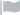Ardian R. 1 0 The relationship of numerator to denominator in research depends on the measurement of the study, which is usually a ratio measurement. For example, if you were measuring the number of clients your particular business handles per month, the numerator is the number of clients divided by a denominator of a monthly clientele average.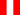Erick V. 0 0 Efectivamente podemos entender que los cambios internos y externos del mercado a la que las empresas están sujetas marcaran claramente el aumento o disminución de la rentabilidad de la empres el riesgo no se puede eliminar pero si mantener un riesgo controlado basado en una planificacion adecuada
• Text Version